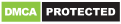# Math games, Mathematics

Genres
Version
5.2.0
Developer
-
Requires
Android 4.2+
Updated
2020-10-01Math games
Test your mind, skill and speed in the fight with time.
There are mathematical methods in just a 2 – 3 seconds multiply 11*83, 104*108, square 75, take the square root of 4489, divide 33126 by 5 and many others.
This application – a game and a guide which includes the most effective mathematical methods of mental arithmetic.

Brain games is based on principles of cognitive psychology to help you practice different mental skill: memory, attention, speed, reaction, concentration, logic and more.
Cool math Games is a brain-training, where logic & thinking & math meet fun ,enjoyment, amusement and lighthearted pleasure. In our Cool math Games App We will See How To play together. So Kids who play our Math Games learn multiplication, fractions, addition, geometry, algebra, problem solving and more!
Cool math games teach you multiply, divide, subtract and square at lightning fast speed.
Math games is a brain gym to reach their full potential.
Increase the result and feel confident in the standard tests: iq test, GRE, GMAT, ACT, MCAT, etc.
Cool math is rest and training. Spend your free time usefully! Good luck!

Maths Tricks:
– subtraction (minus);
– multiplication (multiplication games);
– division (inequality);
– exponentiation (square, cube);
– power of two;
– square numbers ending in 5;
– tough multiplication;
– square root (root);
– percentage (percentage calculator);
– multiplication table.

Math games:
– 2 modes and 16 different types of mathematical tasks (separate operations, combinations thereof or all at once);
– rating system of evaluation and graphs of results;
– auto update due to which there are new – lessons and exercises;
– translated to 7 Languages.

Train, play and have fun! Mathematics – it’s just! Let’s go!
bugs fixed

Recommended for you

You may also like